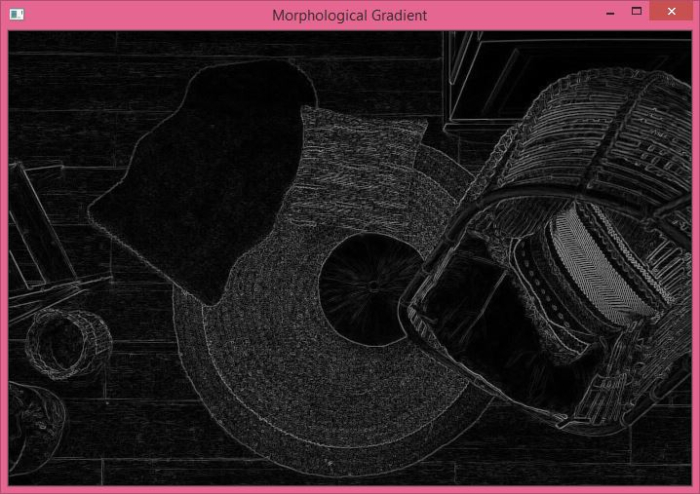# How to compute the morphological gradient of an image using OpenCV in Python?

The morphological gradient is computed as the difference between the dilation and erosion of an image. We use cv2.morphologyEx() method to compute the morphological gradients. Morphological gradient is used in segmentation, edge detection and to find the outline of an object.

## Syntax

Here is the syntax used for this method −

cv2.morphologyEx(img, op, kernel)


Where,

• img − The original input image.

• op − Type of morphological operation. We use cv2.MORPH_GRADIENT.

• kernel − The kernel. We can define the kernel as a numpy matrix of all ones of dtye uint8.

## Steps

You can use the following steps to compute the morphological gradients of an image −

Import the required library. In all the following Python examples, the required Python library is OpenCV. Make sure you have already installed it.

import cv2


img = cv2.imread('floor.jpg', 0)


Compute the morphological gradient using cv2.morphologyEx(). Pass the kernel of desired size.

gradient = cv2.morphologyEx(img, cv2.MORPH_GRADIENT, kernel)


Display the gradient image using cv2.imshow() method.

cv2.imshow("Morphological Gradient", gradient)
cv2.waitKey()
cv2.destroyAllWindows()


Let's have a look at some examples for better understanding.

## Example 1

In this Python example, we compute the morphological gradient of the input image using a 2×2 kernel.

# import required libraries
import cv2
import numpy as np

# define the kernel
kernel = np.ones((2,2),np.uint8)

cv2.waitKey()
cv2.destroyAllWindows()


## We will use the following image as the Input File for this program.## Output

When you run the above Python program, it will produce the following output window −## Example 2

In this example, we compute the morphological gradient of the input image using three different kernels− 2×2, 3×3 and 5×5 kernels.

import cv2
import numpy as np

kernel1 = np.ones((2,2),np.uint8)

kernel2 = np.ones((3,3),np.uint8)

kernel3 = np.ones((5,5),np.uint8)

cv2.waitKey(0)
cv2.destroyAllWindows()


## We will use the following image as the Input File for this program −## Output

When you execute the above program, it will produce three output windows. Three different windows correspond to the three different morphological gradient images using different kernel sizes. Notice the differences among the three outputs.Updated on: 27-Sep-2022

462 Views